# Rise Time: What is it? (Equation And How To Calculate It)

Contents

## What is Rise Time?

Rise time is defined as the time taken for a signal to cross from a specified low value to a specified high value. In analog and digital electronics, the specified lower value and specified higher value are 10% and 90% of the final or steady-state value. So the rise time is typically defined as how long it takes for a signal to go from 10% to 90% of its final value.

The rise time is an essential parameter in analog and digital systems. It describes the time taken for the output to rise from one level to another in an analog system, which has many real-world implications. The rise time tells us how long a signal spends in the intermediate state between two valid logic levels in a digital system.

In control theory, the rise time is defined as a time taken for the response to rising from X% to Y% of its final value. The value of X and Y vary on the type of system.

The rise time for underdamped second-order systems is 0% to 100%, for critically damped systems it is 5% to 95%, and for overdamped systems it is 10% to 90%.

## Rise Time Equation

For the calculation in time domain analysis, we consider the first-order system and second-order system.

So, to calculate the formula for rise time, we consider first-order and second-order systems.

### Rise Time of a First Order System

The first-order system is considered by the following closed-loop transfer function.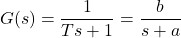In the transfer function, T is defined as a time constant. The time-domain characteristics of the first-order system are calculated in terms of time constant T.

Now, assume that the reference input of the closed-loop system is a unit step function. And it is defined in terms of Laplace transform as;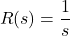So, the output signal will be defined as;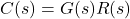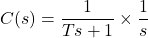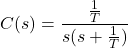Solve this equation using partial fraction;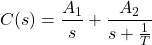Now, find the values of A1 and A2;For s=0;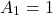For s=-1/T;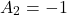Therefore,Taking inverse Laplace;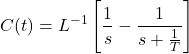Now, we calculate the rise time between 10% and 90% of the final value.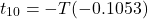Similarly;Now, for rise time tr;### Rise Time of a Second-Order System

In a second-order system, the rise time is calculated from 0% to 100% for the underdamped system, 10% to 90% for the over-damped system, and 5% to 95% for the critically damped system.

Here, we will discuss the calculation of rise time for a second-order system. And the equation for a second-order system is;The rise time is denoted by tr.Where,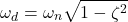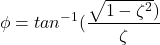Therefore, the final formula of rise time is;## How to Calculate Rise Time?

### First-Order System

For example, find the rise time of a first-order system. The transfer function of a first-order system is shown in the equation below.Compare the transfer function with the standard form of transfer function.So; a=2 and b=5;

The rise time equation for a first order system is;### Second-Order System

Find the rise time of a second-order system with a natural frequency of 5 rad/sec and a damping ratio of 0.6.The equation of rise time for second order system is;Now, we need to find the value of ф and ωd.Now, for ωd,Put these values in the equation of rise time;## Why is Rise Time 10% to 90%?

To calculate the rise time, it is not compulsory that we need to measure the time between 10% to 90%.

But in most cases, the rise time is calculated between these values.

We use these values because the signals may have very different waveforms in the very first and last portions of their final values.

For example, take the switching pattern below:

This was at a value of approximately zero for some time before rising up and reaching its final value.

It would not be appropriate to calculate the “rise time” from when the value was at zero, as this would not be representative of the time taken for the signal to rise during this intermediate state (clearly there was some trigger that occurred at the start of Tr).

On the tail end, we use 90% instead of 100% because often signals will never reach their final value.

Similar to how a logarithmic graph looks, it will never quite reach 100%, with the gradient of the graph decreasing over time.

So to summarise: switching devices have different switching patterns at the starting and ending stages.

But during the transition between these stages, all devices have a similar rise pattern. And measuring 10% to 90% of this transition typically gives a fair representation of the rise time across a wide range of devices.

Hence, in most conditions, we calculate the rise time between 10% and 90%.

## Rise Time vs Fall Time

Fall time is defined as a time taken by a signal to fall (decrease) from a specified value (X) to another specified value (Y).

In most cases, the upper specified value (X) is 90% of the peak value and the lower specified value of 10% of the peak value. A diagram illustrating fall time is shown below.

So in a sense the fall time can be considered the inverse of the rise time, in terms of how it is calculated.

But it is important to underscore that the fall time is not necessarily equal to the rise time.

Unless you have a symmetrical wave (such as a sine wave), the rise time and fall time are independent.

And there is no generalized relationship between the rise time and fall time. Both quantities play a vital role for signal analysis in control systems and digital electronics.

## Rise Time and Bandwidth

To measure the signal practically, we use an oscilloscope. If we know the signal’s rise time, we can find the signal’s bandwidth for testing.

This will help to choose an oscilloscope with greater or equal bandwidth. And it will give accurate display results in the oscilloscope.

If we know the signal’s rise time, we can find how much the oscilloscope will slow down the signal and add to its rise time.

The relation between bandwidth (BW) and rise time (tr) is expressed as the formula below.The above formula assumes that the rise time is measured in the range of 10% to 90% of the final value.

The convenient units of bandwidth are MHz or GHz and for rise time μs or ns.

If an oscilloscope’s input amplifiers have a simple frequency response, the numerator 0.35 gives an accurate result.

But many oscilloscopes have faster roll-off to give a flatter frequency response in the passband. In this condition, the numerator increased to 0.45 or more.

For example, when a square wave is displayed on an oscilloscope, it has a 10-90% rise time of 1ns. What will be the approximate bandwidth of the oscilloscope?

By substituting these numbers into the formula above,Want To Learn Faster? 🎓
Get electrical articles delivered to your inbox every week.
No credit card required—it’s 100% free.